Refer to our Texas Go Math Grade 5 Answer Key Pdf to score good marks in the exams. Test yourself by practicing the problems from Texas Go Math Grade 5 Lesson 13.4 Answer Key Weight.

Essential Question
How can you compare and convert customary units of weight?
To convert from a larger unit of weight to a smaller unit of weight, multiply.
To convert from a smaller unit of weight to a larger unit of weight, divide

Unlock the Problem

Hector’s school is having a model rocket competition. To qualify, each rocket must weigh 4 pounds or less. Hector’s unpainted rocket weighs 62 ounces. What is the weight of the most paint he can use for his model rocket to qualify for entry?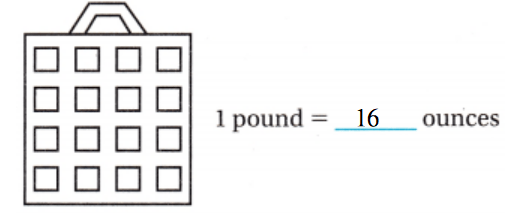• What weight does Hector need to convert?
The weight does Hector needed to convert is: Pound

• After Hector converts the weight, what does he need to do next?
After Hector converted the weight,
He had to find the amount of paint he can use for his model rocket to qualify for entry

Use a strip diagram to write an equation.

Step 1
Convert 4 pounds to ounces.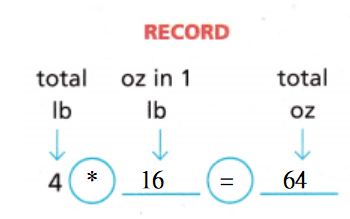Step 2
Subtract the rocket’s weight from the total ounces a rocket can weigh to qualify.
64 – 62 = 2
So, the weight of the paint can be at most 2 ounces for Hector’s model rocket to qualify for entry.

Math Talk
Mathematical Processes
How did you choose which operation to use to change from pounds to ounces? Explain.
We know that,
1 pound = 16 ounces
Now,
From the above relationship,
We can observe that
“Pound” is a larger unit and “Ounce” is a smaller unit
Now,
We know that,
When converting from a large quantity to a small quantity, we will multiply
Hence, from the above,
We can conclude that
The operation that used to change from pounds to ounces is: Multiplication

Example
The rocket boosters for a U.S. space shuttle weigh 1,292,000 pounds each when the shuttle is launched. How many tons does each rocket booster weigh?

Use mental math to convert pounds to tons.

Step 1
Decide which operation to use.
Since pounds are smaller than tons,
I need to divide the number of pounds by 2,000.Step 2
Break 2,000 into two factors that are easy to divide by mentally.
2,000 = 1,000 × 2
Step 3
Divide 1,292,000 by the first factor. Then divide the quotient by the second factor.
1,292,000 ÷ 2,000 = 646
1,292 ÷ 2 = 646
So,
Each rocket booster weighs 646 tons when launched.

Share and Show

Question 1.
Use the picture to complete each equation.
a. 1 pound = ___ ounces
b. 2 pounds = ___ ounces
c. 3 pounds = __ ounces
d. 4 pounds = ___ ounces
e. 5 pounds = ___ ounces
The given table is:Now,
From the given table,
We can observe that
1 pound = 16 ounces
So,
2 pounds = 2 × 16 ounces
= 32 ounces
3 pounds = 3 × 16 ounces
= 48 ounces
4 pounds = 4 × 16 ounces
= 64 ounces
5 pounds = 5 × 16 ounces
= 80 ounces
Hence, from the above.
We can conclude that
1 pound = 16 ounces
2 pounds = 32 ounces
3 pounds = 48 ounces
4 pounds = 64 ounces
5 ounces = 80 ounces

Convert.

15 lb = ______ oz
The given unit is: Pound
The converted unit must be: Ounce
Now,Now,
From the given table,
We can observe that
1 lb = 16 oz
So,
15 lb = 15 × 16 oz
= 240 oz
Hence, from the above,
We can conclude that
15 lb = 240 oz

Question 3.
3 T = ___ lb
The given unit is: Ton
The converted unit must be: Pound
Now,Now,
From the given table,
We can observe that
1 T = 2,000 lb
So,
3 T = 3 × 2,000 lb
= 6,000 lb
Hence, from the above,
We can conclude that
3 T = 6,000 lb

Question 4.
320 oz = __ lb
The given unit is: Ounce
The converted unit must be: Pound
Now,Now,
From the given table,
We can observe that
1 oz = $$\frac{1}{16}$$ lb
So,
320 oz = 320 × $$\frac{1}{16}$$ lb
= 20 lb
Hence, from the above,
We can conclude that
320 oz = 20 lb

Math Talk
Mathematical Processes
Explain how you can compare 11 pounds to 175 ounces mentally?
We know that,
1 pound = 16 ounces
So,
11 pounds = 11 × 16 ounces
= 176 ounces
So,
According to the given information,
176 ounces > 175 ounces
Hence, from the above,
We can conclude that
11 pounds is greater than 175 ounces

Problem Solving

23 lb = ___ oz
The given unit is: Pound
The converted unit must be: Ounce
Now,Now,
From the given table,
We can observe that
1 lb = 16 oz
So,
23 Pounds = 23 × 16
= 368 Ounces
Hence, from the above,
We can conclude that
23 lb = 368 oz

Question 6.
6T = __ lb
The given unit is: Ton
The converted unit must be: Pound
Now,Now,
From the given table,
We can observe that
1 Ton = 2,000 Pounds
So,
6 Tons = 6 × 2,000 Pounds
= 12,000 Pounds
Hence, from the above,
We can conclude that
6 T = 12,000 lb

Question 7.
144 oz = __ lb
The given unit is: Ounce
The converted unit must be: Pound
Now,Now,
From the given table,
We can observe that
1 Ounce = $$\frac{1}{16}$$ Pounds
So,
144 Ounces = 144 × $$\frac{1}{16}$$
= 9 Pounds
Hence, from the above,
We can conclude that
144 oz = 9 lb

Question 8.
15T = ___ lb
The given unit is: Ton
The converted unit must be: Pound
Now,Now,
From the given table,
We can observe that
1 Ton = 2,000 Pounds
So,
15 T = 15 × 2,000 Pounds
= 30,000 Pounds
Hence, from the above,
We can conclude that
15 T = 30,000 lb

Question 9.
352 oz = ___ lb
The given unit is: Ounce
The converted unit must be: Pound
Now,Now,
From the given table,
We can observe that
1 oz = $$\frac{1}{16}$$ lb
So,
352 oz = 352 × $$\frac{1}{16}$$ pounds
= 22 pounds
Hence, from the above,
We can conclude that
352 oz = 22 lb

18 lb = __ oz
The given unit is: Pound
The converted unit must be: Ounce
Now,Now,
From the given table,
We can observe that
1 lb = 16 oz
So,
18 Pounds = 18 × 16
= 288 Ounces
Hence, from the above,
We can conclude that
18 lb = 288 oz

Use Symbols Compare. Write <, >, or =.

Question 11.
130 oz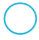8 lb
The given units are: Ounce, Pound
Now,
The given table is:Now,
From the given table,
We can observe that
1 Pound = 16 Ounces
So,
8 Pounds = 8 × 16 Ounces
= 128 Ounces
So,
130 oz > 128 oz
Hence, from the above,
We can conclude thatQuestion 12.
16T32,000 lb
The given units are: Ton, Pound
Now,
The given table is:Now,
From the given table,
We can observe that
1 Ton = 2,000 Pounds
So,
16 Tons = 16 × 2,000 Pounds
= 32,000 Pounds
So,
32,000 lb = 32,000 lb
Hence, from the above,
We can conclude that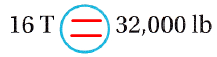Question 13.
14 lb229 oz
The given units are: Pound, Ounce
Now,
The given table is:Now,
From the given table,
We can observe that
1 Pound = 16 Ounces
So,
14 Pounds = 14 × 16 Ounces
= 224 Ounces
So,
224 oz < 229 oz
Hence, from the above,
We can conclude that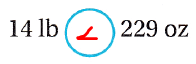Write Math Explain how you can use mental math to compare 7 pounds to 120 ounces.
We know that,
1 Pound = 16 Ounces
So,
7 Pounds = 7 × 16
= 112 Ounces
So,
According to the given information,
112 Ounces < 120 Ounces
Hence, from the above,
We can conclude that
7 Pounds is smaller than 120 Ounces

Problem Solving

Question 15.
H.O.T. Multi-Step Kia wants to have 4 pounds of munchies for her party. She has 36 ounces of popcorn and wants the rest to be pretzel sticks. How many ounces of pretzel sticks does she need to buy?
It is given that
Kia wants to have 4 pounds of munchies for her party. She has 36 ounces of popcorn and wants the rest to be pretzel sticks
Now,
We know that,
1 Pound = 16 Ounces
Now,
According to the given information,
The number of ounces of pretzel sticks does Kia need to buy = (The total number of pounds of munchies) – (The number of ounces of popcorn)
= (4 × 16) – 36
= 64 – 36
= 28 Ounces
Hence, from the above,
We can conclude that
The number of ounces of pretzel sticks does Kia need to buy is: 28 Ounces

Question 16.
H.O.T. Multi-Step Kevin uses 36 ounces of dried apples and 18 ounces of dried cranberries to make a fruit snack. He plans to sell the snack in $$\frac{1}{2}$$-pound containers. How many containers will he fill? Will any fruit snacks be left over?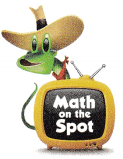It is given that
Kevin uses 36 ounces of dried apples and 18 ounces of dried cranberries to make a fruit snack. He plans to sell the snack in $$\frac{1}{2}$$-pound containers
So,
The total amount of fruit snacks = (The amount of dried apples) + (The amount of dried cranberries)
= 36 + 18
= 54 Ounces
Now,
We know that,
1 pound = 16 Ounces
1 Ounce = $$\frac{1}{16}$$ Pounds
So,
The total amount of fruit snacks = $$\frac{54}{16}$$
= 3 R 6
= 3 Pounds 6 Ounces
Now,
The number of containers will Kevin fill = 54 Ounces ×  2
= 108 Ounces
= $$\frac{108}{16}$$
= 6 R 12
Hence, from the above,
We can conclude that
The number of containers will Kevin fill is: 6
The number of fruit snacks that will be leftover is: 12 Ounces

Question 17.
An elephant’s brain weighs around 11 pounds, while a human’s brain weighs about 48 ounces. How many more ounces does an elephant’s brain weigh than a human’s brain?
(A) 37 ounces
(B) 128 ounces
(C) 4 ounces
(D) 592 ounces
It is given that
An elephant’s brain weighs around 11 pounds, while a human’s brain weighs about 48 ounces
Now,
We know that,
1 Pound = 16 Ounces
So,
The difference of Ounces between an elephant’s brain and a human’s brain = (11 × 16) – 48
= 176 – 48
= 128 Ounces
Hence, from the above,
We can conclude that
The number of more ounces does an elephant’s brain weigh than a human’s brain is: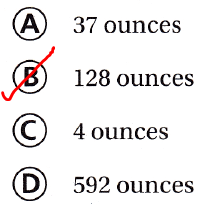Texas Go Math Grade 5 Answer Key Pdf Lesson 13.4 Question 18.
Use Symbols Which of the following inequalities is correct?
(A) 63 ounces > 4 pounds
(B) 40 pounds < 600 ounces (C) 3.5 tons > 5,000 pounds
(D) 200 pounds < 3,000 ounces
The inequalities that are correct is:Question 19.
Multi’Step A 9-pound block of butter is delivered each morning to a restaurant. A recipe for 1 pot of soup calls for 6 ounces of butter. Using all of the butter, how many pots of soup can the restaurant make each day?
(A) 24 pots of soup
(B) 18 pots of soup
(C) 11 pots of soup
(D) 4 pots of soup
It is given that
A 9-pound block of butter is delivered each morning to a restaurant. A recipe for 1 pot of soup calls for 6 ounces of butter
So,
The number of pots of soup the restaurant can make each day = $$\frac{9 × 16}{6}$$
= 24 pots of soup
Hence, from the above,
We can conclude that
The number of pots of soup the restaurant can make each day is:Texas Test Prep

Question 20.
Carlos used 32 ounces of walnuts in a muffin recipe. How many pounds of walnuts did Carlos use?
(A) 8 pounds
(B) 4 pounds
(C) 2 pounds
(D) 1 pound
It is given that
Carlos used 32 ounces of walnuts in a muffin recipe
Now,
We know that,
1 Ounce = $$\frac{1}{16}$$ Pounds
So,
According to the given information,
The number of pounds of walnuts did Carlos used = 32 × $$\frac{1}{16}$$ Pounds
= 2 Pounds
Hence, from the above,
We can conclude that
The number of pounds of walnuts did Carlos used is: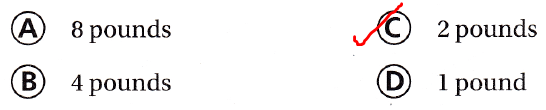### Texas Go Math Grade 5 Lesson 13.4 Homework and Practice Answer Key

Convert.

Question 1.
160 oz = __ lb
The given unit is: Ounce
The converted unit must be: Pound
Now,Now,
From the given table,
We can observe that
1 Ounce = $$\frac{1}{16}$$ Pounds
So,
160 Ounces = 160 × $$\frac{1}{16}$$ Pounds
= 10 Pounds
Hence, from the above,
We can conclude that
160 oz = 10 lb

Question 2.
5 T = __ lb
The given unit is: Ton
The converted unit must be: Pound
Now,Now,
From the given table,
We can observe that
1 Ton = 2,000 Pounds
So,
5 Tons = 5 × 2,000 Pounds
= 10,000 Pounds
Hence, from the above,
We can conclude that
5 T = 10,000 lb

Question 3.
16 lb = ___ oz
The given unit is: Pound
The converted unit must be: Ounce
Now,Now,
From the given table,
We can observe that
1 Pound = 16 Ounces
So,
16 Pounds = 16 × 16 Ounces
= 256 Ounces
Hence, from the above,
We can conclude that
16 lb = 256 oz

Question 4.
12 T = __ lb
The given unit is: Ton
The converted unit must be: Pound
Now,Now,
From the given table,
We can observe that
1 Ton = 2,000 Pounds
So,
12 Tons = 12 × 2,000 Pounds
= 24,000 Pounds
Hence, from the above,
We can conclude that
12 T = 24,000 lb

Question 5.
128 oz = __ lb
The given unit is: Ounce
The converted unit must be: Pound
Now,Now,
From the given table,
We can observe that
1 Ounce = $$\frac{1}{16}$$ Pounds
So,
128 Ounces = 128 × $$\frac{1}{16}$$ Pounds
= 8 Pounds
Hence, from the above,
We can conclude that
128 oz = 8 lb

Question 6.
26 lb = __ oz
The given unit is: Pound
The converted unit must be: Ounce
Now,Now,
From the given table,
We can observe that
1 Pound = 16 Ounces
So,
26 Pounds = 26 × 16 Ounces
= 416 Ounces
Hence, from the above,
We can conclude that
26 lb = 416 oz

Compare. Write <, >, or =.

Question 7.
140 oz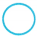9 lb
The given units are: Ounce, Pound
Now,
The given table is:Now,
From the given table,
We can observe that
1 Pound = 16 Ounces
So,
9 Pounds = 9 × 16 Ounces
= 144 Ounces
So,
140 oz < 144 oz
Hence, from the above,
We can conclude thatQuestion 8.
7T15,000 lb
The given units are: Ton, Pound
Now,
The given table is:Now,
From the given table,
We can observe that
1 Ton = 2,000 Pounds
So,
7 Tons = 7 × 2,000 Pounds
= 14,000 Pounds
So,
14,000 lb < 15,000 lb
Hence, from the above,
We can conclude that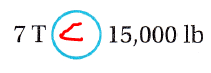Question 9.
208 oz13 lb
The given units are: Ounce, Pound
Now,
The given table is:Now,
From the given table,
We can observe that
1 Pound = 16 Ounces
So,
13 Pounds = 13 × 16 Ounces
= 208 Ounces
So,
208 oz = 208 oz
Hence, from the above,
We can conclude that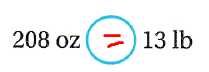Question 10.
23 lb365 oz
The given units are: Pound, Ounce
Now,
The given table is:Now,
From the given table,
We can observe that
1 Pound = 16 Ounces
So,
23 Pounds = 23 × 16 Ounces
= 368 Ounces
So,
368 oz > 365 oz
Hence, from the above,
We can conclude that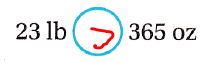20 T40,000 lb
The given units are: Ton, Pound
Now,
The given table is:Now,
From the given table,
We can observe that
1 Ton = 2,000 Pounds
So,
20 Ton = 20 × 2,000 Pounds
= 40,000 Pounds
So,
40,000 lb = 40,000 lb
Hence, from the above,
We can conclude thatQuestion 12.
19 lb298 oz
The given units are: Pound, Ounce
Now,
The given table is:Now,
From the given table,
We can observe that
1 Pound = 16 Ounces
So,
19 Pounds = 19 × 16 Ounces
= 304 Ounces
So,
304 oz > 298 oz
Hence, from the above,
We can conclude thatQuestion 13.
Explain how you can use mental math to compare 175 ounces to 11 pounds.
We know that,
1 Pound = 16 Ounces
So,
11 Pounds = 11 × 16
= 176 Ounces
So,
175 oz < 176 oz
Hence, from the above,
We can conclude that
175 Ounces is less than 11 Pounds

Problem Solving

Question 14.
Peanuts in bulk bins at the grocery store cost $0.30 per ounce. How much would 2 pounds of peanuts cost? Answer: It is given that Peanuts in bulk bins at the grocery store cost$0.30 per ounce
Now,
We know that,
1 Pound = 16 Ounces
So,
According to the given information,
The cost of 2 pounds of peanuts = (The number of pounds of peanuts) × (The number of Ounces for each Pound) × (The cost of peanuts for each Ounce)
= 2 × 16 × $0.30 = 32 ×$0.30
= $9.6 Hence, from the above, We can conclude that The cost of 2 pounds of peanuts is:$9.6

Question 15.
Pecans in bulk bins at the grocery store cost $0.65 per ounce. How much would you spend if you bought 1 pound 8 ounces of peanuts and 2 pounds of pecans? Answer: It is given that Pecans in bulk bins at the grocery store cost$0.65 per ounce.
Now,
We know that,
1 Pound = 16 Ounces
Now,
From Exercise 14,
Peanuts in bulk bins at the grocery store cost $0.30 per ounce. So, According to the given information, The total amount of money spent = (Te total cost of peanuts) + (the total cost of peacons) = (16 + 8) ×$0.30 + (2 × 16) × $0.65 =$7.2 + $20.8 =$28
Hence, from the above,
We can conclude that
The total amount of money spent is: \$28

Texas Test Prep

Lesson Check

Question 16.
Greg’s cocker spaniel weighs 32 pounds. His kitten weighs 15 ounces. How many more ounces does the cocker spaniel weigh than the kitten?
(A) 256 ounces
(B) 241 ounces
(C) 497 ounces
(D) 512 ounces
It is given that
Greg’s cocker spaniel weighs 32 pounds. His kitten weighs 15 ounces
Now,
We know that,
1 Pound = 16 Ounces
So,
The number of more ounces does the cocker spaniel weigh than the kitten = (32 × 16) – 15
= 512 – 15
= 497 Ounces
Hence, from the above,
We can conclude that
The number of more ounces does the cocker spaniel weigh than the kitten is: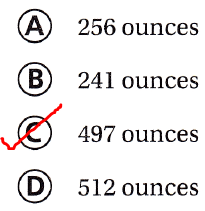Question 17.
The snack machine at the ballpark contains 48 ounces of potato chips. How many pounds of chips are in the snack machine?
(A) 4 pounds
(B) 2 pounds
(C) 6 pounds
(D) 3 pounds
It is given that
The snack machine at the ballpark contains 48 ounces of potato chips
Now,
We know that,
1 Ounce = $$\frac{1}{16}$$ Pounds
So,
According to the given information,
The number of pounds of chips are in the snack machine = 48 × $$\frac{1}{16}$$
= 3 Pounds
Hence, from the above,
We can conclude that
The number of pounds of chips are in the snack machine is: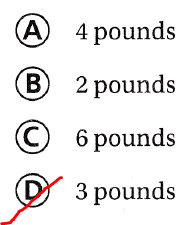Question 18.
A bag of corn chips weighs 8 ounces. How many bags of chips weigh 12 pounds?
(A) 24
(B) 6
(C) 16
(D) 48
It is given that
A bag of corn chips weighs 8 ounces
Now,
We know that,
1 Pound = 16 Ounces
So,
8 Ounces = 8 × $$\frac{1}{16}$$
= $$\frac{1}{2}$$ Pounds
So,
According to the given information,
The number of bags of chips that weigh 12 pounds = 12 ÷$$\frac{1}{2}$$
= 12 × 2
= 24 bags
Hence, from the above,
We can conclude that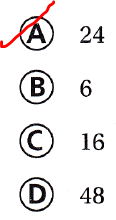Question 19.
Which expression could be used to convert 86,000 pounds to tons?
(A) 86,000 ÷ 1,000
(B) 86,000 × 1,000
(C) 86,000 × 2,000
(D) 86,000 ÷ 2,000
We know that,
1 Pound = $$\frac{1}{2,000}$$ Tons
Hence, from the above,
We can conclude that
The expression that could be used to convert 86,000 pounds to tons is:Question 20.
Which of the following inequalities is correct?
(A) 12.5 pounds > 200 ounces
(B) 490 ounces > 30 pounds
(C) 6 tons < 10,000 pounds
(D) 8,000 pounds < 4 tons
The following inequalities that are correct is:Question 21.
A car weighs 2 tons. How many ounces equal 2 tons?
(A) 64,000
(B) 4,000
(C) 32,000
(D) 6,400
It is given that
A car weighs 2 tons
Now,
We know that,
1 Ton = 2,000 Pounds
1 Pound = 16 Ounces
So,
According to the given information,
2 tons = 2 × 2,000 × 16
= 64,000 Ounces
Hence, from the above,
We can conclude that
The number of Ounces that equals to 2 Tons is: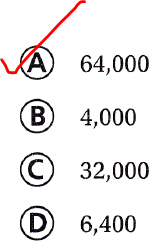Question 22.
Multi-Step Eight baseballs weigh 40 ounces. How many pounds do 16 baseballs weigh?
(A) 5 pounds
(B) 4 pounds
(C) 20 pounds
(D) 80 pounds
It is given that
Eight baseballs weigh 40 ounces
Now,
We know that,
1 Ounce = $$\frac{1}{16}$$ Pounds
So,
The total weight of 16 baseballs = (The weight of 8 baseballs) × 2
= 40 ×2
= 80 Ounces
So,
The total weight of 16 baseballs in Pounds = 80 ×$$\frac{1}{16}$$
= 5 Pounds
Hence, from the above,
We can conclude that
The total weight of 16 baseballs in Pounds is:Question 23.
Multi-Step A football weighs 14 ounces. A carton of footballs weighs 7 pounds. How many footballs are in a carton?
(A) 12
(B) 8
(C) 7
(D) 4
It is given that
A football weighs 14 ounces. A carton of footballs weighs 7 pounds
Now,
We know that,
1 Pound = 16 Ounces
So,
The weight of a carton in Ounces = 7 × 16
= 112 Pounds
So,
According to the given information,
The number of footballs that are in a carten = $$\frac{112}{14}$$
= 8 footballs
Hence, from the baove,
We can conclude that
The number of footballs that are in a carton is: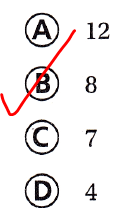Scroll to Top